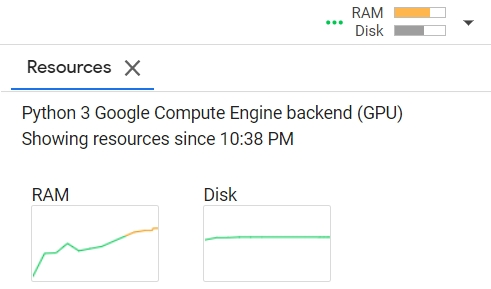# RAM issue with semi-supervised CNN model training

Hey Guys,
I’m trying to training a binary classifier by transfer learning on EfficientNet. Since I have lots of unlabeled data, I use semi-supervised method to generate multiple “pseudo labeled” data before the model go through each epoch.

Since Colab has its limits of RAM usage, I delete some large variables(like numpy arrays, dataset, dataloader…) in each loop, however the RAM still increase in every loop like the picture shown below.

Below is my Training loop which consists of 3 main structure: semi-supervised, training loop, validation loop. I’m not sure which step cause the RAM to keep increase in each epoch.

(1) semi-supervised

``````for epoch in range(n_epochs):
print(f"[ Epoch | {epoch + 1:03d}/{n_epochs:03d} ]")
if do_semi:
model.eval()
dataset_0 = []
dataset_1 = []

logits = model(img.to(device))
probs = softmax(logits)

# Filter the data and construct a new dataset.
for i in range(len(probs)):
p = probs[i].tolist()
idx = p.index(max(p))
if p[idx] >= threshold:
if idx == 0:
dataset_0.append(img[i].numpy().reshape(128, 128, 3))
else:
dataset_1.append(img[i].numpy().reshape(128, 128, 3))
# stratified sampling with labels
len_0, len_1 = len(dataset_0), len(dataset_1)
print('label 0: ', len_0)
print('label 1: ', len_1)

# since there may be RAM memory error, restrict to 1000
if len_0 > 1000:
dataset_0 = random.sample(dataset_0, 1000)
if len_1 > 1000:
dataset_1 = random.sample(dataset_1, 1000)

if len_0 == len_1:
pseudo_x = np.array(dataset_0 + dataset_1)
pseudo_y = ['0' for _ in range(len(dataset_0))] + ['1' for _ in range(len(dataset_1))]

elif len_0 > len_1:
dataset_0 = random.sample(dataset_0, len(dataset_1))
pseudo_x = np.array(dataset_0 + dataset_1)
pseudo_y = ['0' for _ in range(len(dataset_0))] + ['1' for _ in range(len(dataset_1))]

else:
dataset_1 = random.sample(dataset_1, len(dataset_0))
pseudo_x = np.array(dataset_0 + dataset_1)
pseudo_y = ['0' for _ in range(len(dataset_0))] + ['1' for _ in range(len(dataset_1))]

if len(pseudo_x) != 0:
new_dataset = CustomTensorDataset(pseudo_x, np.array(pseudo_y), 'pseudo')
else:
new_dataset = []
# print how many pseudo label data added
print('Total number of pseudo labeled data are added: ', len(new_dataset))
# release RAM
dataset_0 = None
dataset_1 = None
pseudo_x  = None
pseudo_y  = None
del dataset_0, dataset_1, pseudo_x, pseudo_y
gc.collect()
# Turn off the eval mode.
model.train()

concat_dataset = ConcatDataset([train_set, new_dataset])